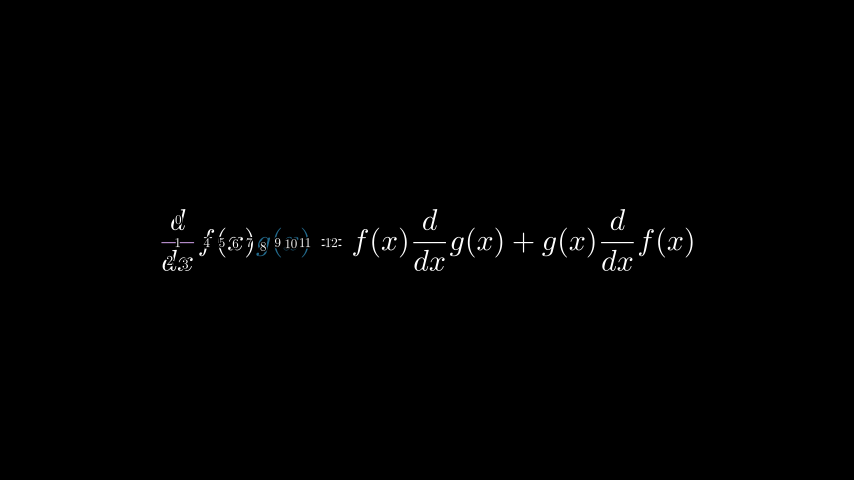# debug#

Debugging utilities.

Functions

index_labels(mobject, label_height=0.15, background_stroke_width=5, background_stroke_color='#000000', **kwargs)[source]#

Returns a `VGroup` of `Integer` mobjects that shows the index of each submobject.

Useful for working with parts of complicated mobjects.

Parameters
• mobject (Mobject) – The mobject that will have its submobjects labelled.

• label_height (float) – The height of the labels, by default 0.15.

• background_stroke_width – The stroke width of the outline of the labels, by default 5.

• background_stroke_color – The stroke color of the outline of labels.

• kwargs – Additional parameters to be passed into the :class`~.Integer` mobjects used to construct the labels.

Examples

Example: IndexLabelsExample```from manim import *

class IndexLabelsExample(Scene):
def construct(self):
text = MathTex(
"\\frac{d}{dx}f(x)g(x)=",
"f(x)\\frac{d}{dx}g(x)",
"+",
"g(x)\\frac{d}{dx}f(x)",
)

#index the fist term in the MathTex mob
indices = index_labels(text)

text.set_color(PURPLE_B)
text[8:12].set_color(DARK_BLUE)